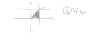# Double integral question

• math_04
The integral is the sum of the inverse of each function multiplied by its inverse.In summary, the homework statement is trying to find the inverse of each function.

## Homework Statement

Consider the integral shown in the sketch. Sketch the region of integration and express the integral with the reverse order of integration and evaluate it leaving your answer in surd form

## The Attempt at a Solution

I shaded the area of integration but I am not sure whether it is the right area. How do I know which area of integration to use? And secondly, when you choose your x limits, do you draw a horizontal line that passes through the y- axis and through the sketched functions? Likewise when you choose your y limits, do you draw a vertical line that passes through the x-axis and through the sketched functions? Also just wondering whether the shaded area is only half of the region to integrate? Maybe I could only integrate that half area and double the answer?

#### Attachments

•integral sketch.JPG
7.2 KB · Views: 445
Please try to improve sketches of problems.

I think the double integral is

$$\int^1_0\,\int^1_{\sqrt{x}}\,\sqrt{1+y^3}\,dy dx$$

Ok,

draw a straight line parallel to y-axis that goes through your currently shaded region.

now, they are saying that y limits from going from sqrt(x) to 1

so, pick the starting point on the vertical line and the ending point.\

Astronuc, yea that is the right integral. But now, i don't know whether my shaded region is the right one?

Oh and rootX, don't u find out the limits after you know which area to integrate under?

math_04 said:
Oh and rootX, don't u find out the limits after you know which area to integrate under?

Limits are given. Those dy goes from sqrt(x) to 1 and dx from 0 to 1

More correctly, y goes from sqrt(x) to 1 and x goes from 0 to 1.

Now, the two graphs, y= sqrt(x) (or x= y^2) and x= 1 intersect at (0,0) and (1,1).

If you integrate with respect to x first and then y, the limits of integration on y must be numbers. What values does y go between? In other words, what are the smallest and largest values of y? Those are the limits of integration on y.

Now, for each y (draw a horizontal line on your graph), what values of y does x lie between (the lower and upper limits for x may be functions of y).